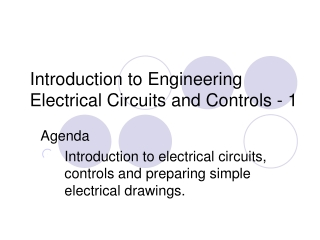Download PresentationIntroduction to Engineering Electrical Circuits and Controls - 1

# Introduction to Engineering Electrical Circuits and Controls - 1 - PowerPoint PPT PresentationDownload Presentation## Introduction to Engineering Electrical Circuits and Controls - 1

- - - - - - - - - - - - - - - - - - - - - - - - - - - E N D - - - - - - - - - - - - - - - - - - - - - - - - - - -
##### Presentation Transcript

1. Introduction to EngineeringElectrical Circuits and Controls - 1 Agenda Introduction to electrical circuits, controls and preparing simple electrical drawings.

2. Agenda • Discuss basic concepts for electrical circuits and controls. • Learn how to read and create simple electrical drawings.

3. Electrical Drawings • Electrical drawings provide a complete description of the electrical circuitry for a product. • The purpose of a circuit diagram is to clearly show how components are connected electrically. This is not the same as showing where components are physically, or how they have been laid out on a circuit board. • Standard symbols are used.

4. Symbols for Basic Components in a Circuit resistor inductor capacitor voltage source current source switch

5. Example: Single Use Camera Flash Circuit

6. Creating Electrical Circuit Diagram for a Device - Flashlight (Switch) S (Resistive Load) R (Battery) V (Current) I actual object electrical drawing Electrons flow from (-) to (+); by convention, current is said to flow in the opposite direction.

7. Ohm’s Law V = IR The voltage change V (volts) across any resistive load is equal to the product of the current I (amps) and the resistance R (Ohms).

8. Basic Relationship – Power Law P = IV Power dissipated P (watts) is equal to product of the current I (amps) and voltage V (volts)

9. Example 1 – Instructor Example R = 12 ohms 120 V i = ? Current I = V/R = 120 V/12 Ohms = 10 amps Power P = V I= 120 V * 10 Amps = 1200 Watts

10. Example 2 – Student Example R = 24 Ohms 240 V I = ? I = P =

11. Example 3 – Resistance of Light Bulbs • Without doing any calculations, which light bulb has the lowest resistance? • 75 W bulb at 120 V • 150 W bulb at 120 V

12. Example 3 – Resistance of Light Bulbs, calculate and compare Calculate the resistance using Power Law and Ohms Law. Use: I = P / V (P = VI) & R = V / I • Some groups do 75 W bulb • Other groups do 150 W bulb • Compare Results

13. Assignment #28 • Do problem set 1 on electrical circuits found in the assignment packet.